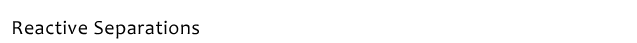Reaction Kinetics

The Starks Mechanism points to an organic phase reaction that, for most reactions, is rate controlling. A second order rate expression (first order in product formation) for making a final product R-X in a batch process is:

d [R-X]org = k2[Qorg] [R-Y]org
dt

where

[R-X] = Final Product Concentration, gmol/lit
k2 = Second order rate constant, lit-gmol/min
[Q] = Catalyst concentration in the organic phase, gmol/lit
[R-Y] = Organic Phase Soluble Reactant gmol/lit

This results from the mole balance for R- that is illustrated by:

 d[R-X] = - d [R-Y] dt dt

The following plots show the conversion profile for making final products benzyl phenyl ether and benzyl cyanide. Derivation of the kinetic rate constant for benzyl cyanide is shown by plotting -ln(1-conv) vs. time as shown in the graph. The slope of the line represents the psuedo first order rate constant in units of reciprocal minutes. Dividing this constant by the catalyst concentration gives the second order velocity constant k2 in lit/(gmol-min).